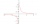# Pool

If water flows into the pool by two inlets, fill the whole for 8 hours. The first inlet filled pool 6 hour longer than second. How long pool take to fill with two inlets separately?

Result

t1 =  19.54 h
t2 =  13.54 h

#### Solution:

$\dfrac{ 1 }{ t_{ 1 } } +\dfrac{ 1 }{ t_{ 2 } } = \dfrac{ 1 }{ 8 } \ \\ t_{ 2 } = t_{ 1 } - 6 \ \\ 1/t_{ 1 } + 1/(t_{ 1 }-6) = 1/8 \ \\ \ \\ \ \\ 8 \cdot \ (x-6) + 8 \cdot \ x = (x-6) \cdot \ x \ \\ -x^2 +22x -48 = 0 \ \\ x^2 -22x +48 = 0 \ \\ \ \\ a = 1; b = -22; c = 48 \ \\ D = b^2 - 4ac = 22^2 - 4\cdot 1 \cdot 48 = 292 \ \\ D>0 \ \\ \ \\ x_{1,2} = \dfrac{ -b \pm \sqrt{ D } }{ 2a } = \dfrac{ 22 \pm \sqrt{ 292 } }{ 2 } = \dfrac{ 22 \pm 2 \sqrt{ 73 } }{ 2 } \ \\ x_{1,2} = 11 \pm 8.54400374532 \ \\ x_{1} = 19.5440037453 \ \\ x_{2} = 2.45599625468 \ \\ \ \\ \text{ Factored form of the equation: } \ \\ (x -19.5440037453) (x -2.45599625468) = 0 \ \\ t_{ 1 }>0 \ \\ t_{ 1 } = x_{ 1 } = 19.544 \doteq 19.544 = 19.54 \ \text { h }$

Checkout calculation with our calculator of quadratic equations.

$t_{ 2 }>0 \ \\ t_{ 2 } = t_{ 1 } - 6 = 19.544 - 6 = \dfrac{ 677 }{ 50 } = 13.54 = 13.54 \ \text { h }$

Leave us a comment of this math problem and its solution (i.e. if it is still somewhat unclear...):Math student
1/t1+1/(t1-10)=1/18
multiply each term by18(t1)(t1-10)
that results in
18(t1-10)+18t1=t1(t1)(t1)-10t1
using the quadratic formula results in t1=-49.6 and 3.63

10 months ago  2 LikesDr Math
right side of equation is wrong - should be t1*(t1-10) = t12 - 10*t1 now t13-10t1Math student
the problems seems to have changed - - - t2 is now equal t1-6

therefore 1/t1+1/(t1-6)=1/18
multiplying each term by18(t1)(t1-6) ==== 18(t1-6)+18t1=t1(t1-6), simplifying further 18t1-108+18t1=t12-6t1
or 0=t12-6t1-18t1+108
graphing y=18(t1-6)+18t1-t1(t1-6) results in t1=39.25 hours and t2=39.25-6=33.25 hours (same as your NEW answer!!!!#### Following knowledge from mathematics are needed to solve this word math problem:

Looking for help with calculating harmonic mean? Looking for a statistical calculator? Looking for help with calculating roots of a quadratic equation? Do you have a linear equation or system of equations and looking for its solution? Or do you have quadratic equation? Tip: Our volume units converter will help you with the conversion of volume units.

## Next similar math problems:

1. The hemisphereThe hemisphere container is filled with water. What is the radius of the container when 10 liters of water pour from it when tilted 30 degrees?
2. LogicA man can drink a barrel of water for 26 days, woman for 48 days. How many days will a barrel last between them?
3. Three-day tripThe cyclist on three-day trip travel 30% of the total route on the first day, 3/5 of the rest on the second day and 35 km on the third day. How many kilometers did travel cyclists each day and how many?
4. DiscountLadies sweater was twice discounted. First by 11%, then by 11% of the new price. Its final price was 100 €. Determine the original price of sweater.
5. Land boundaryThe land has the shape of a right triangle. The hypotenuse has a length of 30m. The circumference of the land is 72 meters. What is the length of the remaining sides of the land boundary?
6. Sides of right angled triangleOne leg is 1 m shorter than the hypotenuse, and the second leg is 2 m shorter than the hypotenuse. Find the lengths of all sides of the right-angled triangle.
7. Before yesterdayHe merchant adds a sale sign in his shop window to the showed pair of shoes in the morning: "Today by p% cheaper than yesterday. " After a while, however, he decided that the sign saying: "Today 62.5% cheaper than the day before yesterday". Determine the n
8. Secret treasureScouts have a tent in the shape of a regular quadrilateral pyramid with a side of the base 4 m and a height of 3 m. Determine the radius r (and height h) of the container so that they can hide the largest possible treasure.
9. Equation 23Find value of unknown x in equation: x+3/x+1=5 (problem finding x)
10. Geography testsOn three 150-point geography tests, you earned grades of 88%, 94%, and 90%. The final test is worth 250 points. What percent do you need on the final to earn 93% of the total points on all tests?
11. Medians in right triangleIt is given a right triangle, angle C is 90 degrees. I know it medians t1 = 8 cm and median t2 = 12 cm. .. How to calculate the length of the sides?
12. Faces diagonalsIf the diagonals of a cuboid are x, y, and z (wall diagonals or three faces) respectively than find the volume of a cuboid. Solve for x=1.2, y=1.7, z=1.45
13. Trapezoid MOThe rectangular trapezoid ABCD with right angle at point B, |AC| = 12, |CD| = 8, diagonals are perpendicular to each other. Calculate the perimeter and area of ​​the trapezoid.
14. BonusGross wage was 527 EUR including 16% bonus. How many EUR were bonuses?
15. BeerAfter three 10° beers consumed in a short time, there is 5.6 g of alcohol in 6 kg adult human blood. How much is it per mille?
16. ClockHow many times a day hands on a clock overlap?
17. ServerCalculate how many average minutes a year is a webserver is unavailable, the availability is 99.99%.